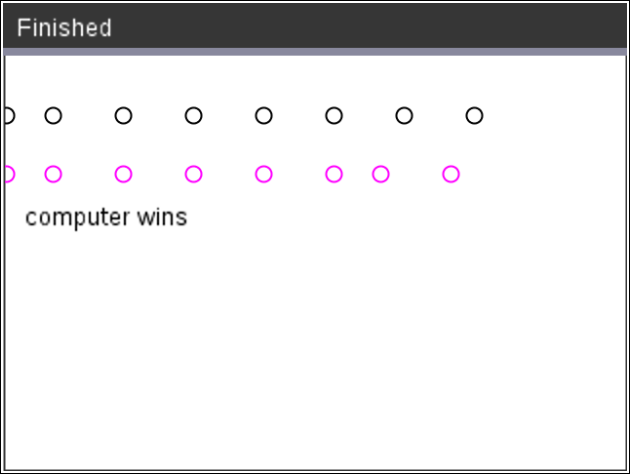•• ##### Device
•TI-Nspire™ CX II
•TI-Nspire™ CX II CAS
• ##### Software

TI-Nspire™ CX
TI-Nspire™ CX CAS

5.2

# Explorations with Coding: Ready, Set... Solve!

by Texas Instruments#### Objectives

• Use functions to generate strings and integers
• Use the randint() function to generate random integers.
• Use the TI-Draw library to plot circles
• Use loops to repeat code
• Use if statements to make selections
• Use the sleep function to animate the plot
• Practice solving multi-step equations

#### About the Lesson

In this lesson, students will use python to create a fast-paced game to help students practice solving multi-step equations.  If the player’s math skills are on point, they will out run the computer. Make too many mistakes, and the calculator will win the race.

The program will ask a series of questions.  If the user answers a question correctly, the user will “run” fast.  If the user answers a question incorrect, they slow down.  The computer answer runs each leg of the race using random probability.